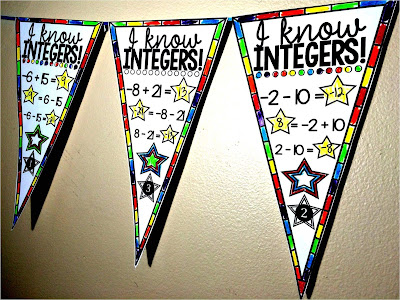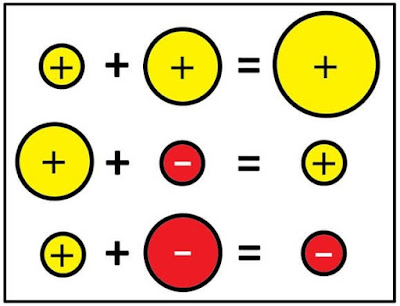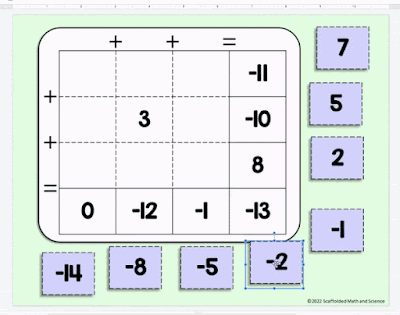## Pages

### Integer Operations Graphic Organizer

With integer multiplication and division, the rules are clean and concrete. A negative times a negative is literally the "opposite of a negative" and the rules are pretty simple to remember. With addition and subtraction the rules are a whole lot looser. For students who like things nice and clear, integers can be a real sticking point.

As part of my graduate thesis I made this integer operations manipulative that reduced my students' errors with integers by 62%. It allows students to "see" how the numbers relate to one another and helps concrete thinkers find success.

Yesterday a teacher asked if I could also come up with a graphic organizer for adding and subtracting integers that was like the one I had made for solving equations. I pulled together this graphic organizer. It works about the same as the manipulative and is geared towards students who don't need as much support as the manipulative offers.

How to use the graphic organizer:

2: Now look at the integers' signs. Both positive? One negative, one positive? If so, which is "heavier"? (ie farther from zero).

3: Follow the column that matches the integers you have.

Example:

For a problem like...
5 - 14

5 + -14

2: The "heavier" number is negative because -14 is father from zero than 5 is. So we follow the 3rd column.

3: "subtract"* (find 14 - 5) and keep the -

5 + -14 = -9

*Since we're actually subtracting the absolute values of the numbers, I added a footnote on the graphic organizer. I will also ask my students, "how much farther from zero is 14 than 5?" when completing this last step.

More integers:Integers math pennant

A fun activity to practice integer addition and subtraction is this integers math pennant. Students add and subtract integers, then personalize their pennants to become colorful classroom décor.Integer addition and subtraction posters

There are some additional visuals for integer addition and subtraction in this visual integers post. These posters with different sized red and yellow dots are based on a post by Don Steward, who allowed me to make the printables based on his post.Adding integers puzzles

Here's a newer set of 3 adding integers puzzles that come in 2 versions-- PDF printable and digital in Google Slides (above). Ideas for differentiating the puzzles are inside the PDF.

1.Please could you make multiplication and division posters for integers. The addition and subtraction ones are awesome!!!

1.Hi Vicki! If you search my blog for "integers visuals" a post will come up with red and yellow dots. In there is a new poster for integer multiplication and division. It's towards the bottom.

2.